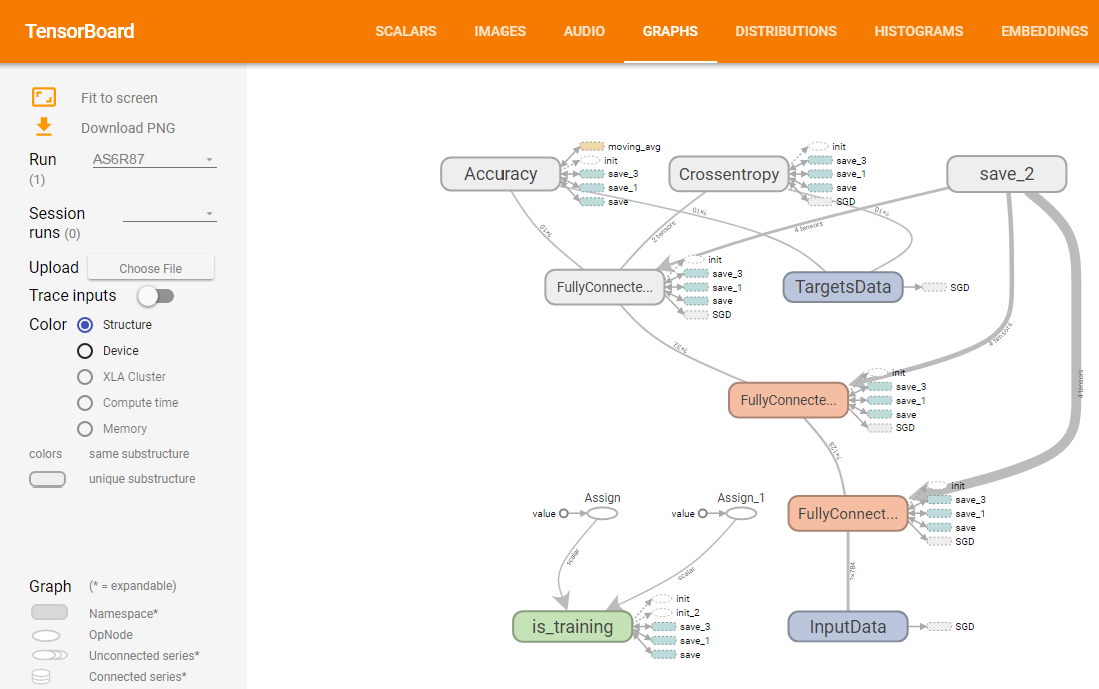# プログラミング ＋ アカデミック + 何か面白いこと

2. 0 view

## Visualization of Neural Network and its Training using TensorboardPocket

# Introduction

Sometimes I guess you think you want to visualize Neural Networks and see learning curve immediately when you use tensorflow and tflearn.

I found a good tool. It’s called tensorboard. I’ll show you how to use it.

You can try samples easily in this article with following Docker image.

# Environment

I used tflearn to make model easily.

• OS : Ubuntu 16.04
• python : 3.5.2
• tensorflow : 1.1.0
• tfLearn : 0.3
• tensorboard : 1.0.0a6

# Install tensorboard

It’s super easy. Only pip install command is only you need to run. But, looks I couldn’t run tensorboard in virtualenv. So, if you meet a problem with virtualenv, please try it out of virtualenv.

```pip install tensorboard
```

# Sample Neural Network

I’ll create a model to recognize hand-written digits using MNIST dataset.

If you don’t have numpy, tensorflow and tflearn, please install with pip install command.

I’ll create a very simple model. Number of input layer units is 784(28 x 28 pixel). There are 2 hidden layers. One of them is 128 units and the other’s number of units is 32. Number of output layer’s unit is 10 to output digits from 0 to 9.Actual code is following.

```import numpy as np
import tensorflow as tf
import tflearn
import tflearn.datasets.mnist as mnist

X_train, y_train, X_test, y_test = mnist.load_data(one_hot=True)

# 2. Build a NN Model
tf.reset_default_graph()
net = tflearn.input_data([None, X_train.shape]) # Input Layer
net = tflearn.fully_connected(net, 128, activation='ReLU') # Hidden Layer 1
net = tflearn.fully_connected(net, 32, activation='ReLU') # Hidden Layer 2
net = tflearn.fully_connected(net, 10, activation='softmax') # Output Layer
net = tflearn.regression(net, optimizer='sgd', learning_rate=0.01, loss='categorical_crossentropy')
model = tflearn.DNN(net, tensorboard_verbose=3)

# 3. Traning
model.fit(X_train, y_train, validation_set=0.1, show_metric=True, batch_size=100, n_epoch=20)

# 4. Testing
predictions = np.array(model.predict(X_test)).argmax(axis=1)
actual = y_test.argmax(axis=1)
test_accuracy = np.mean(predictions == actual, axis=0)
print("Test accuracy: ", test_accuracy)
```

Result of my trial is around 91% accuracy.

# See The Model and Traning Status on Tensorboard

tflearn automatically outputs log files into /tmp/tflearn_logs. Tensorboard can use the log file.

Tensorboard is WebUI and it’s default port is 6006. You can launch it with following command.

```tensorboard --logdir='/tmp/tflearn_logs' --port=6006
```

You can see the results with accessing to like http://localhost:6006 by browser.

GRAPHS tab shows model topology and training information.SCALARS tab shows training status. If you train the model sometimes, you can see multiple results on the tab.# Take a Look at a Model on Jupyter Notebook

If you want to take a look at a Neural Network model which you created on Jupyter Notebook, you can use tfgraphviz.

You can install tfgraphviza with just running pip install.

```pip install tfgraphviz
```

I couldn’t output a model which I created using tflearn. So, in this time I used tensorflow. I used this article’s sample .

```import numpy as np
import tensorflow as tf

tf.reset_default_graph()

# Creating input and correct result data
x_data = np.random.rand(100).astype(np.float32)
y_data = x_data * 0.1 + 0.3

# Build network
W = tf.Variable(tf.random_uniform(, -1.0, 1.0))
b = tf.Variable(tf.zeros())
y = W * x_data + b
loss = tf.reduce_mean(tf.square(y - y_data))
train = optimizer.minimize(loss)

# Output graph with tfgraphviz
tfg.board(tf.get_default_graph())
```

The result is following.This is not very easy to understand at first look. But, you can understand overview.

# Docker image

You can run Jupyter notebook and Tensorboard by following commands. The image has samples.ipynb which has this article’s all codes.

Pocket